# Difference between revisions of "Bradley-Terry model"

The names of R.A. Bradley and M.E. Terry are associated with a model that is widely employed in paired comparisons. In paired comparison experiments, observations are made by presenting pairs of objects to one or more judges. This method is used in experimental situations where objects are judged subjectively (cf. also Paired comparison model). The basic model was presented in [a1]. The model is mostly formulated as follows (see, e.g., [a2]).

The paired comparison experiment hasobjects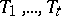withcomparisons of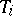and,,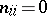,,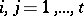. Some of the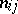may be zero, but the design should be connected, i.e., there must not be any subset of the treatments such that no treatment in this subset is compared with any treatment of the complementary subset. The model postulates the existence of treatment parametersfor(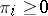),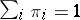, such that the probability of selecting, when compared with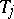, is equal to.

The likelihood method can be used to estimate the parameters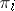(cf. Likelihood equation). If the comparisons are independent, the likelihood functionis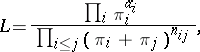whereand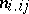is the number of timeshas been preferred towhenandwere compared (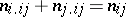). In [a3], large-sample results and the asymptotic distribution of the maximum-likelihood estimators are given. L.R. Ford has described an iterative solution of the likelihood equations (see [a4]). R.J. Beaver has presented, [a5], a weighted least-squares approach to paired comparison models. He has also used a method, described in [a6], which presents a unified approach to the analysis of data resulting from an experiment involving multinomial populations. Thus, general results from weighted least-squares methods can be used. A. Springall (see [a7]) has considered the case where the parameters() are functions of continuous variables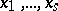. He has formulated a model that is linear in the unknown parameters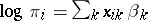. In this case the parameters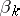have to be estimated. He has given results concerning the asymptotic variance-covariance matrix of the estimators.

A linear model like the one above can be used to answer optimal design questions.